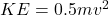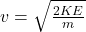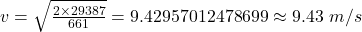## A car is strapped to a rocket (combined mass = 661 kg), and its kinetic energy is 66,120 J. At this time, the rocket runs out of

Question

A car is strapped to a rocket (combined mass = 661 kg), and its kinetic energy is 66,120 J.

At this time, the rocket runs out of fuel and turns off, and the car deploys a parachute to slow down, and the parachute performs 36,733 J of work on the car.

What is the final speed of the car after this work is performed?

in progress 0
6 months 2021-08-07T16:00:32+00:00 1 Answers 3 views 0

9.43 m/s

Explanation:

The law of conservation of energy requires that the total energy before and after transformation must be equal. Kinetic energy of car and rocket combined equals the parachute energy and that of car.

Final energy of car will be 66120-36733=29,387 J

Kinetic energy,and making v the subject of the formula thenWhere m is the mass and v is velocity.

Substituting 29387 J for KE and 661 kg for m then